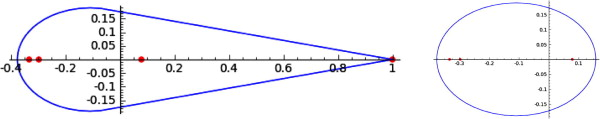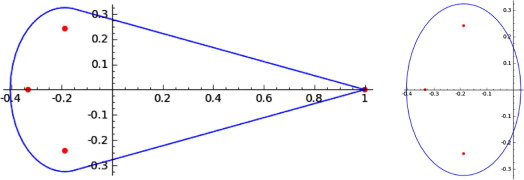# Numerical range of doubly stochastic matrices

## Doubly stochastic matrices

A doubly stochastic matrix $A \in \mathbb{R}^{n \times n }$ is a matrix for which the entries are non-negative while the row and column sums are all equal to one $\sum_{j=1}^{n} a_{ij} = 1 \text{ for } i=1,\ldots,n$ and $\sum_{i=1}^{n} a_{ij} = 1 \text{ for } j=1,\ldots,n$

### Theorem

Let A be $4\times 4$ doubly stochastic matrix. Then, $W(A)$consists of elliptical arcs and line segments if and only if$$:= (A) - 1 + is an eigenvalue of A (multiple, if$$\mu =1$$). If, in addition A - 1 - 3>0 , (A -1 - 3)^2 - (A^A) + 1 +2 + ^2 > 0$$

then $W(A)$ also has corner points at $\mu$ and 1, and thus four flat portions on the boundary. Otherwise, 1 is the only corner point of $W(A)$ and $\partial W(A)$ consists of two flat portions and one elliptical arc.

### Example 1

Consider the doubly stochastic matrix : $A = \begin{pmatrix} 0&1/3&1/4&5/12\ 1/3&0&1/2&1/6\ 1/4&9/32&1/4&1/6\ 5/12&37/96&0&19/96 \end{pmatrix}$

Using above theorem, we compute $\mu = -1/3.$ By calculating the characteristic polynomial and computin the conditions from Theorem we have that one of condition is negative, Theorem 1 states that $\partial W(A)$ has two flat portions and one elliptical arc. Indeed, A reduces unitarily to $(1) \oplus A_1$ for some $3\times 3$ matrix $A_1$, and in Fig. 1 we give $W(A)$, and the horizontal ellipse it contains, $W(A_1)$.Fig. 1 Numerical range of $A$ with two flat portions and an elliptical arc on the boundary, and the ellipse it contains. The eigenvalues of A are indicated by the points.

### Example 2

Consider the doubly stochastic matrix : $A = \begin{pmatrix} 0&1/3&1/4&5/12\ 1/3&0&1/2&1/6\ 1/4&1/8&1/4&3/8\ 5/12&13/24&0&1/24 \end{pmatrix}$ By coincidence, we again compute $\mu = -1/3$, and though the characteristic polynomial again has $\mu$ as a root. The formulas in inequalities on Theorem evaluate to $7/24$ and $59/576$ respectively, so the number of flat portions is still the same. However, Fig. 2 shows that $W(A)$ is the convex hull of a vertical ellipse and the point 1, as opposed to the horizontal ellipse in the previous example. Indeed, the eigenvalues marked in the graph include a complex conjugate pair.Fig. 2 Numerical range $A$ with two flat portions and an elliptical arc on the boundary, and the contained vertical ellipse. The eigenvalues of $A$ are indicated by the points.

This section is created based on  in which we can find more examples also.

1. K. A. Camenga, P. X. Rault, D. J. Rossi, T. Sendova, and I. M. Spitkovsky, “Numerical range of some doubly stochastic matrices,” Applied Mathematics and Computation, vol. 221, pp. 40–47, 2013, [Online]. Available at: https://www.sciencedirect.com/science/article/pii/S0096300313006231.